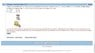# Solving for different masses in pulley system with KE

• jb007

## Homework Statement

So I am stuck on this homework problem. I understand the general direction I have to take, but my algebra and physics aren't good. Here's the problem:
A simple Atwood's machine uses two masses, m1 and m2. Starting from rest, the speed of the two masses is 10.0 m/s at the end of 6.0 s. At that instant, the kinetic energy of the system is 90 J and each mass has moved a distance of 30.0 m. Determine the values of m1 and m2.

## Homework Equations

Wnet = change in KE
total KE of the system = 0.5(m1)v^2 + 0.5(m2)v^2
W = force * distance

## The Attempt at a Solution

My work so far has not really gotten me anywhere, any tips would be very helpful.

First, I saw that the KE of the system would be the KE equation but with m 1 and m 2 , like this: KE = 0.5(m1+m2 )v^2, or 90=0.5(m1+m2 )v^2, or 90=0.5(m1+m2 )100

So then then m1+m2 would be equal to 9/5.

I'm not sure about this next part I've done: The net work is equal to the change in KE. Since the system starts from rest, the initial KE is 0, and the final KE is 90.

So I did: W=0.5(m1+m2 )100. Then using the work equation: FΔX=0.5(m1+m2)100, and since ΔX is equal to 30m: 30F=0.5(m1+m2)100

I tried substituting in the acceleration in order to get the force of the masses, 10/6=5/3m/s/s, so weight 1 = m1*5/3, and weight 2 = m2*5/3.

With these, I know there is supposed to be 2 equations for 2 unknowns, but I can't seem to figure them out. I'm kind of lost at his point.

I've uploaded a picture of the problem. I believe I'm headed in the right direction. Any tips?

#### Attachments

•q11.jpg
28.8 KB · Views: 495
30F=0.5(m1+m2)100
And what is F?
edit: You actually don't need to worry about F; what is the kinetic energy?

I tried substituting in the acceleration in order to get the force of the masses, 10/6=5/3m/s/s, so weight 1 = m1*5/3, and weight 2 = m2*5/3.
This is not right. The weight 1 = m1g and the weight 2 = m2g

And what is F?
edit: You actually don't need to worry about F; what is the kinetic energy?

This is not right. The weight 1 = m1g and the weight 2 = m2g
The total kinetic energy is 90J. So would it be 90=0.5(m1+m2)v^2, which solving for m1+m2 would get me 9/5kg, like I had before. I'm failing to see the connection between this and the force.
I think the net force on m1 should be T-m1g, where T is the tension. And the net force on m2 should be T-m2g? And would T be equal to the sum of the weights of the blocks?

I think the net force on m1 should be T-m1g, where T is the tension. And the net force on m2 should be T-m2g?
Good. Do you know a relationship between the net force and acceleration?

Good. Do you know a relationship between the net force and acceleration?
Ok, so then by F=ma, the net force for object 1 would be T-m1g=m1a, and the net force for object 2 would be T-m2g = m2a.
Then since a is the same for each object, (T-m1g)/m1 = (T-m2g)/m2?

Ok, so then by F=ma, the net force for object 1 would be T-m1g=m1a, and the net force for object 2 would be T-m2g = m2a.
Then since a is the same for each object, (T-m1g)/m1 = (T-m2g)/m2?
Right, but there's no need to eliminate the acceleration a
Use the two equations to eliminate the tension T
Then you will you have your second relationship between m1 and m2